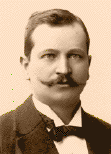Home

Lecture Notes

PHY 521/321:
Condensed Matter Physics I

Prof. S. Teitel stte@pas.rochester.edu ---- Spring 2008

## Lecture Notes

The lecture note files correspond roughly to the material presented in a given day's lecture. But you may on occassion find the end of one day's lecture at the start of the file for the next day's lecture, so please look there if you think there might be something missing.

• Lecture 1 - Introduction, metals

• Lecture 2 - Drude model, dc conductivity, Hall effect

• Lecture 3 - ac conductivity, EM wave propagation in metals, thermal conduction and Weidemann-Franz law

• Lecture 4 - Sommerfeld model, ideal gas of fermions, Fermi energy, density of states, ground state total energy, pressure and bulk modulus

• Lecture 5 - Fermi gas at finite temperature, chemical potential and specific heat of electron gas

• Lecture 6 - Transport properties of Sommerfeld model, Pauli paramagnetism

• Lecture 7 - Electrons in a magnetic field, Landau levels, flux quantum

• Lecture 8 - Density of states in a magnetic field, van Hove singularities, variation of Fermi energy

• Lecture 9 - Ground state energy in a magnetic field, Landau diamagnetism, de Haas - van Alphen oscillations
Some improved notes on Landau diamagnetism can be found here.

• Lecture 10 - Screening of Coulomb interactions in the electron gas, the Thomas-Fermi dielectric function

• Lecture 11 - The Lindhard dielectric function, Friedel (or Rudderman-Kittel) oscillations, Kohn anomaly

• Lecture 11A - The RKKY interaction and spin glasses

• Lecture 12 - Plasma oscillations, Wigner electron crystal

• Lecture 13 - Cooper pairing

• Lecture 14 - Bravais lattices, crystal structures

• Lecture 15 - Reciprocal lattice, X-ray scattering

• Lecture 16 - Preview of electrons in a crystal, X-ray scattering from Bravais lattice with a basis

• Lecture 17 - Electrons in a crystal: qualitative arguments

• Lecture 18 - Electrons in a crystal: boundary conditions and Fourier transforms on a Bravais Lattice, Bloch's theorem, reduced, extended and periodic zone schemes for the band structure, velocity of a Bloch electron

• Lecture 19 - Density of states and van Hove singularities, the weak potential approximation for energy gaps, eigenstates, and velocity near a Bragg plane

• Lecture 20 - Band structure in the weak potential approximation, metals and insulators
The 2D Brillouin Zones in living color!

• Lecture 21 - Bravais lattice with a basis in weak potential approximation, Brillouin Zones and the Fermi surface in the weak potential approximation

• Lecture 22 - The tight binding approximation for band structure

• Lecture 23 - Tight binding band structure of graphene

• Lecture 24 - Wannier functions, spin-orbit interaction, semiclassical equations of motion

• Lecture 25 - Motion in uniform electric and magnetic fields, effective mass, holes

• Lecture 26 - The Hall effect

• Lecture 27 - Lattice vibrations, normal modes, dispersion relations and polarizations

• Lecture 28 - Bohm-Stavier relation for the speed of sound